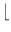Next: General Weighted Matchings ( Up: Graph Algorithms Previous: Bipartite Weighted Matchings and   Contents   Index

# Maximum Cardinality Matchings in General Graphs ( mc_matching )

A matching in a graph G is a subset M of the edges of G such that no two share an endpoint.

An odd-set cover OSC of G is a labeling of the nodes of G with non-negative integers such that every edge of G (which is not a self-loop) is either incident to a node labeled 1 or connects two nodes labeled with the same i, i > = 2.

Let ni be the number of nodes labeled i and consider any matching N. For i, i > = 2, let Ni be the edges in N that connect two nodes labeled i. Let N1 be the remaining edges in N. Then | Ni| < =ni/2and | N1| < = n1 and hencefor any matching N and any odd-set cover OSC.

It can be shown that for a maximum cardinality matching M there is always an odd-set cover OSC withthus proving the optimality of M. In such a cover all ni with i > = 2 are odd, hence the name.

 list MAX_CARD_MATCHING(const graph& G, node_array& OSC, int heur = 0) computes a maximum cardinality matching M in G and returns it as a list of edges. The algorithm (, ) has running time O(nm*(n, m)). With heur = 1 the algorithm uses a greedy heuristic to find an initial matching. This seems to have little effect on the running time of the algorithm. An odd-set cover that proves the maximality of M is returned in OSC. list MAX_CARD_MATCHING(const graph& G, int heur = 0) as above, but no proof of optimality is returned. bool CHECK_MAX_CARD_MATCHING(const graph& G, const list& M, const node_array& OSC) checks whether M is a maximum cardinality matching in G and OSC is a proof of optimality. Aborts if this is not the case.Next: General Weighted Matchings ( Up: Graph Algorithms Previous: Bipartite Weighted Matchings and   Contents   Index